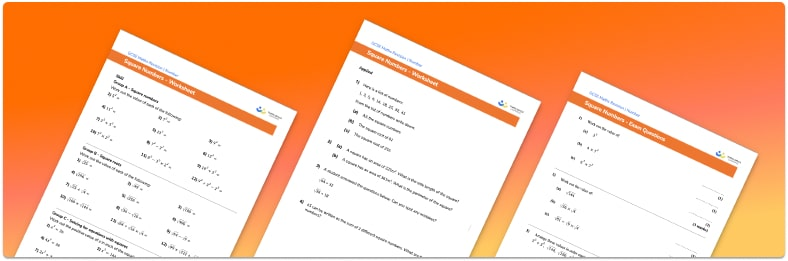# Square Numbers Worksheet• Section 1 of the square numbers  worksheet contains 20+ skills-based square numbers  questions, in 3 groups to support differentiation
• Section 2 contains 3 applied square numbers  questions with a mix of word problems and deeper problem solving questions
• Section 3 contains 3 foundation and higher level GCSE exam style square numbers  questions
• Answers and a mark scheme for all square numbers  questions are provided
• Questions follow variation theory with plenty of opportunities for students to work independently at their own level
• All questions and maths activities created by fully qualified expert secondary maths teachers
• Suitable for GCSE maths revision for AQA, OCR and Edexcel exam boards

• This field is for validation purposes and should be left unchanged.

You can unsubscribe at any time (each email we send will contain an easy way to unsubscribe). To find out more about how we use your data, see our privacy policy.

### Square numbers at a glance

A square number is the result when a number is multiplied by itself. For example, 3 times 3=9 and so 9 is a square number. Square numbers are sometimes called perfect squares. The first 12 square numbers are 1, 4, 9, 16, 25, 36, 49, 64, 81, 100, 121 and 144

The inverse of squaring a number is square rooting a number. The square root of a number is the number that is squared to get that number, so the square root of 9 is 3.

It helps to be confident with times tables when exploring square numbers and square roots.

When squaring a negative number, the answer is always positive. We must therefore be aware that the square root of a number can also have a negative solution, e.g. -4 times -4=16 and so the square root of 16 is equal to 4 and -4.

Also, the square of an integer is always an integer (a whole number) but the square root of an integer may not always be an integer. If the square root of a number is not an integer, then this number is a surd, e.g. the square root of 3 is not an integer and so the square root of 3 is a surd.

Looking forward, students can then progress to additional powers and roots worksheets and other number worksheets, for example a multiplying and dividing decimals worksheet or an order of operations worksheet.For more teaching and learning support on Number our GCSE maths lessons provide step by step support for all GCSE maths concepts.

## Do you have KS4 students who need more focused attention to succeed at GCSE?There will be students in your class who require individual attention to help them succeed in their maths GCSEs. In a class of 30, it’s not always easy to provide.

Help your students feel confident with exam-style questions and the strategies they’ll need to answer them correctly with our dedicated GCSE maths revision programme.

Lessons are selected to provide support where each student needs it most, and specially-trained GCSE maths tutors adapt the pitch and pace of each lesson. This ensures a personalised revision programme that raises grades and boosts confidence.

Find out more